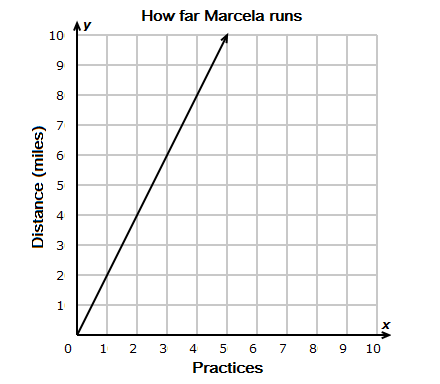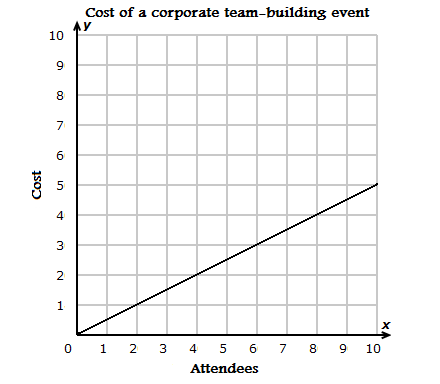Constant of Proportionality Activity

Example 1: This graph shows how the distance Marcela runs depends on the number of track practices she attends.

Example 2: This graph shows how the cost of a corporate team-building event depends on the number of attendees.Practices, x Distance in miles, y yx = k 1 2 21 = ? 2 4 42 = ? 3 6 63 = ? 4 8 84 = ? 5 10 105 = ?

What is the constant of proportionality, k?